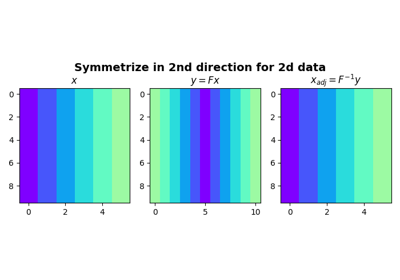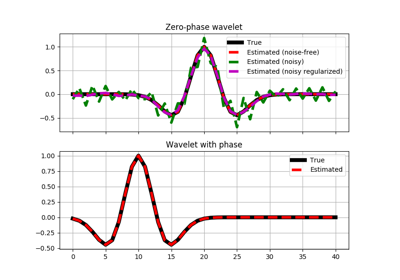# pylops.Symmetrize¶

class pylops.Symmetrize(N, dims=None, dir=0, dtype='float64')[source]

Symmetrize along an axis.

Symmetrize a multi-dimensional array along a specified direction dir.

Parameters: N : int Number of samples in model. Symmetric data has $$2N-1$$ samples dims : list, optional Number of samples for each dimension (None if only one dimension is available) dir : int, optional Direction along which symmetrization is applied dtype : str, optional Type of elements in input array

Notes

The Symmetrize operator constructs a symmetric array given an input model in forward mode, by pre-pending the input model in reversed order.

For simplicity, given a one dimensional array, the forward operation can be expressed as:

$\begin{split}y[i] = \begin{cases} x[i-N],& i\geq N\\ x[N-i],& \text{otherwise} \end{cases}\end{split}$

for $$i=0,1,2,...,2N-2$$, where $$N$$ is the lenght of the input model.

In adjoint mode, the Symmetrize operator assigns the sums of the elements in position $$N-i$$ and $$N+i$$ to position $$i$$ as follows:

$\begin{multline} x[i] = y[N-i]+y[N+i] \quad \forall i=1,2,...,N-1 \end{multline}$

apart from the central sample where $$x = y[N]$$.

Attributes: shape : tuple Operator shape explicit : bool Operator contains a matrix that can be solved explicitly (True) or not (False)

Methods

 __init__(self, N[, dims, dir, dtype]) Initialize this LinearOperator. adjoint(self) Hermitian adjoint. cond(self, \*\*kwargs_eig) Condition number of linear operator. conj(self) Complex conjugate operator div(self, y[, niter]) Solve the linear problem $$\mathbf{y}=\mathbf{A}\mathbf{x}$$. dot(self, x) Matrix-matrix or matrix-vector multiplication. eigs(self[, neigs, symmetric, niter]) Most significant eigenvalues of linear operator. matmat(self, X) Matrix-matrix multiplication. matvec(self, x) Matrix-vector multiplication. rmatvec(self, x) Adjoint matrix-vector multiplication. transpose(self) Transpose this linear operator.

## Examples using pylops.Symmetrize¶SymmetrizeWavelet estimation